## How to Calculate and Solve for Dimensionless Time in Well Testing | The Calculator Encyclopedia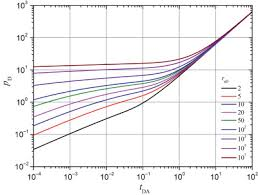The image above represents dimensionless time.

To compute for the dimensionless time, six essential parameters are needed and these parameters are permeability (k), porosity (φ), viscosity (μ), well radius (rw), minimum transient time (t) and total compressibility (CT).

The formula for calculating dimensionless time:

tD = 0.000263kt / φμCTr

Where;

tD = Dimensionless Time
k = Permeability
φ = Porosity
μ = Viscosity
t = Minimum Transient Time
CT = Total Compressibility

Let’s solve an example
Find the dimensionless time when the permeability is 13, porosity is 11.5, viscosity is 10.5, well radius is 24, minimum transient time is 17 with a total compressibility of 28.

This implies that;

k = Permeability = 13
φ = Porosity = 11.5
μ = Viscosity = 10.5
rw = Well Radius = 24
t = Minimum Transient Time = 17
CT = Total Compressibility = 28

tD = 0.000263kt / φμCTr
tD = 0.000263 x 13 x 1711.5 x 10.5 x 28 x 24²
tD = 0.000263 x 13 x 1711.5 x 10.5 x 28 x 576
tD = 0.000263 x 13 x 171947456
tD = 0.0581231947456
tD = 2.9845e-8

Therefore, the dimensionless time is 2.98

Nickzom Calculator – The Calculator Encyclopedia is capable of calculating the dimensionless time.

To get the answer and workings of the dimensionless time using the Nickzom Calculator – The Calculator Encyclopedia. First, you need to obtain the app.

You can get this app via any of these means:

To get access to the professional version via web, you need to register and subscribe for NGN 1,500 per annum to have utter access to all functionalities.
You can also try the demo version via https://www.nickzom.org/calculator

Apple (Paid) – https://itunes.apple.com/us/app/nickzom-calculator/id1331162702?mt=8
Once, you have obtained the calculator encyclopedia app, proceed to the Calculator Map, then click on Petroleum under EngineeringNow, Click on Well Testing under Petroleum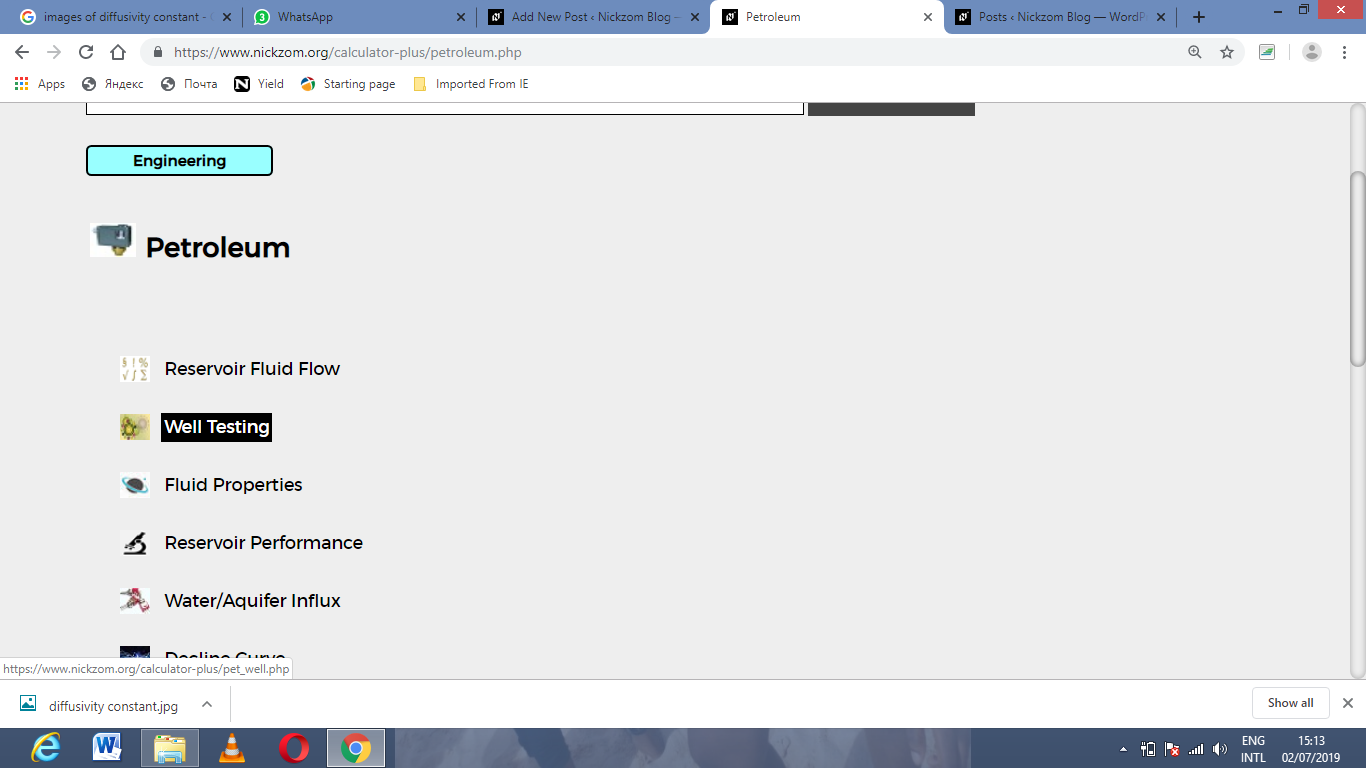Now, Click on Dimensionless Time under Well Testing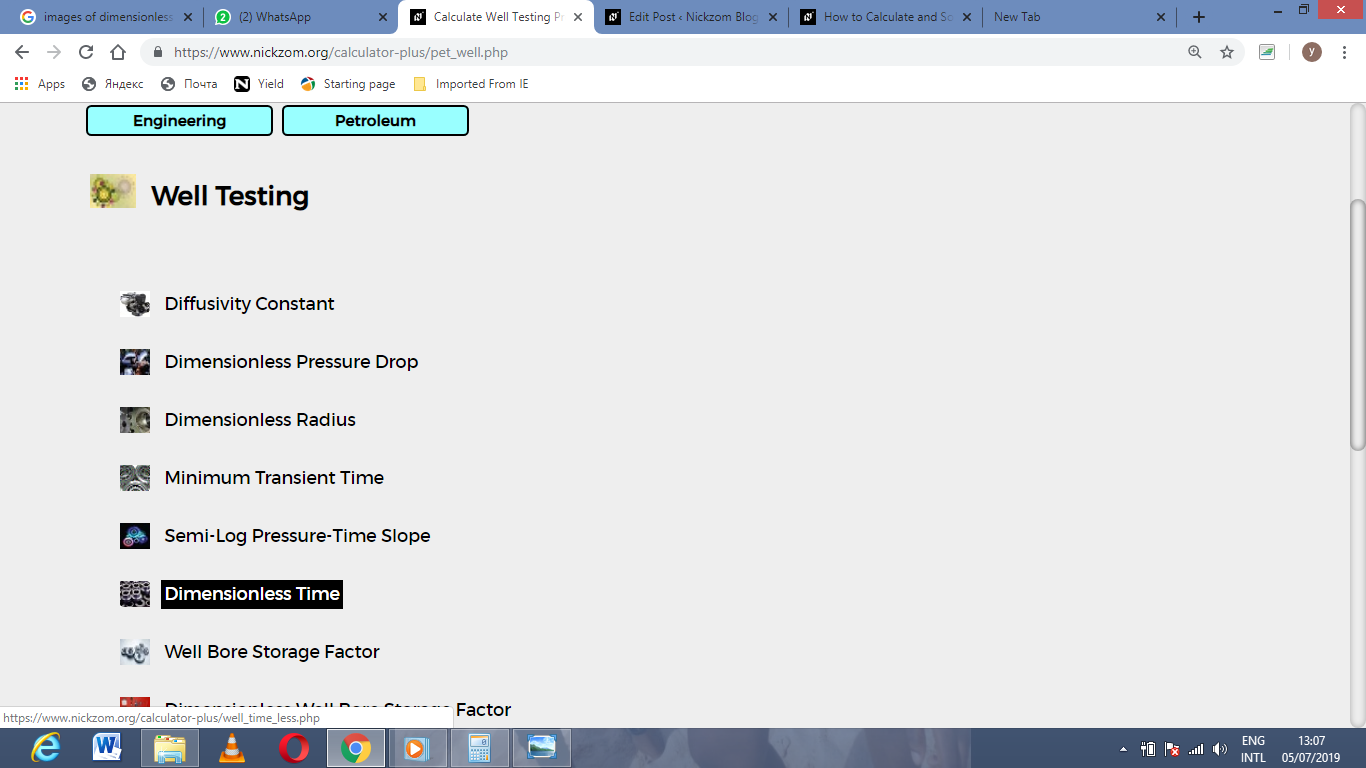The screenshot below displays the page or activity to enter your value, to get the answer for the dimensionless time according to the respective parameter which are the permeability (k), porosity (φ), viscosity (μ), well radius (rw), minimum transient time (t) and total compressibility (CT).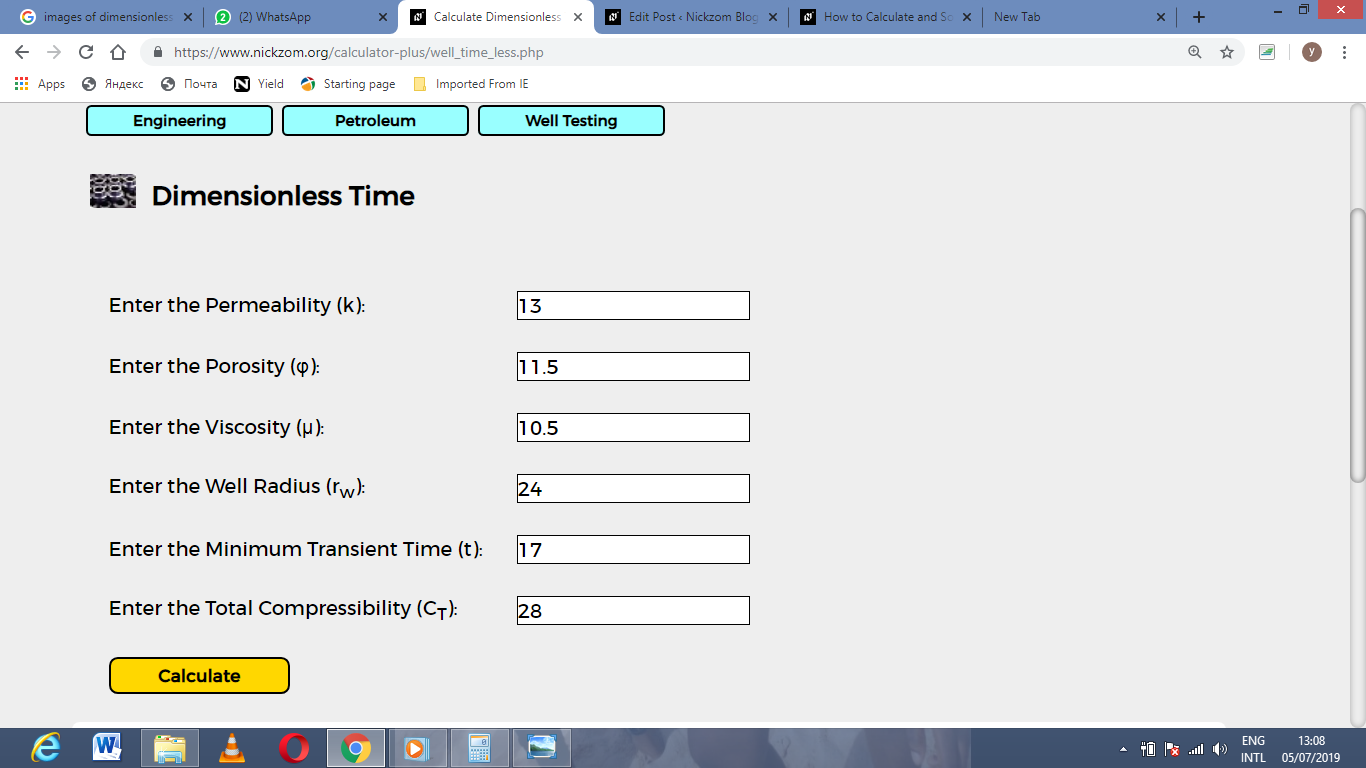Now, enter the value appropriately and accordingly for the parameter as required by the permeability (k) is 13, porosity (φ) is 11.5, viscosity (μ) is 10.5, well radius (rw) is 24, minimum transient time (t) is 17 and total compressibility (CT) is 28.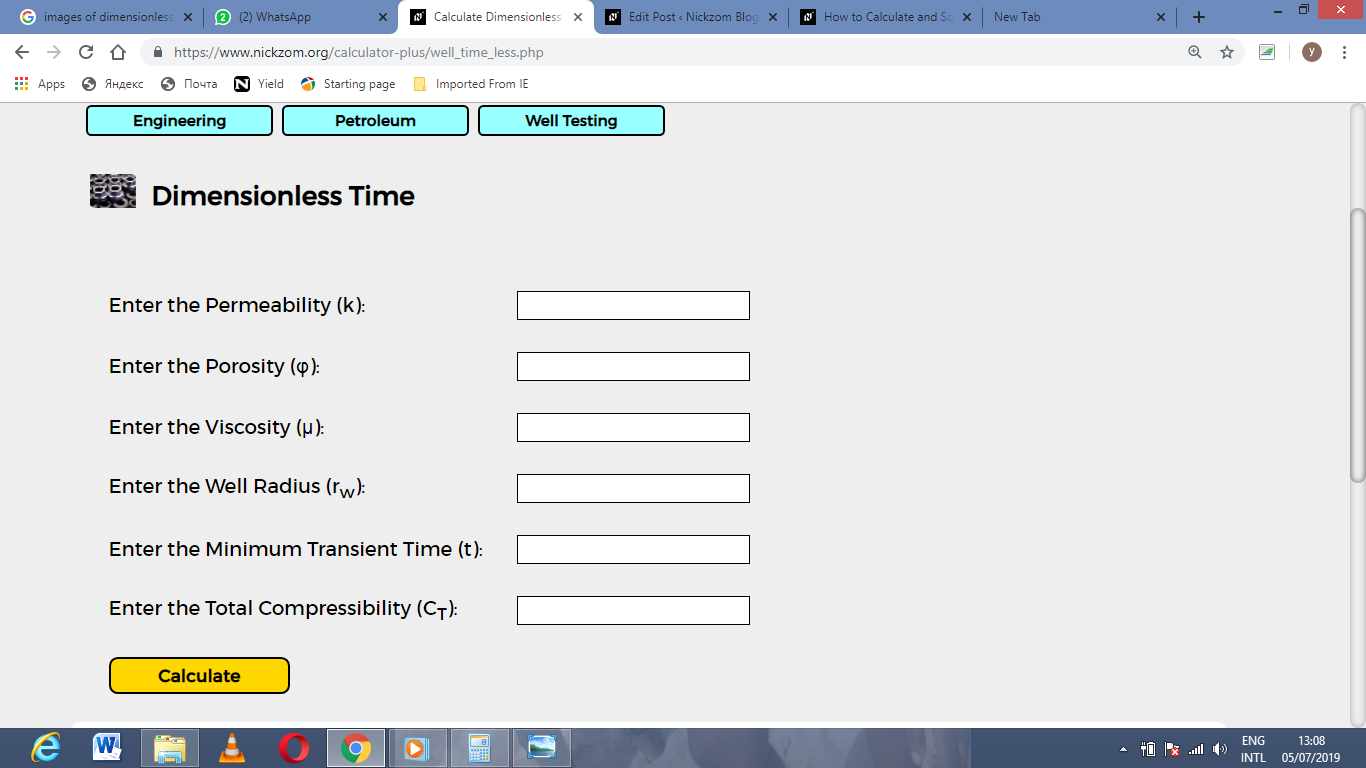Finally, Click on Calculate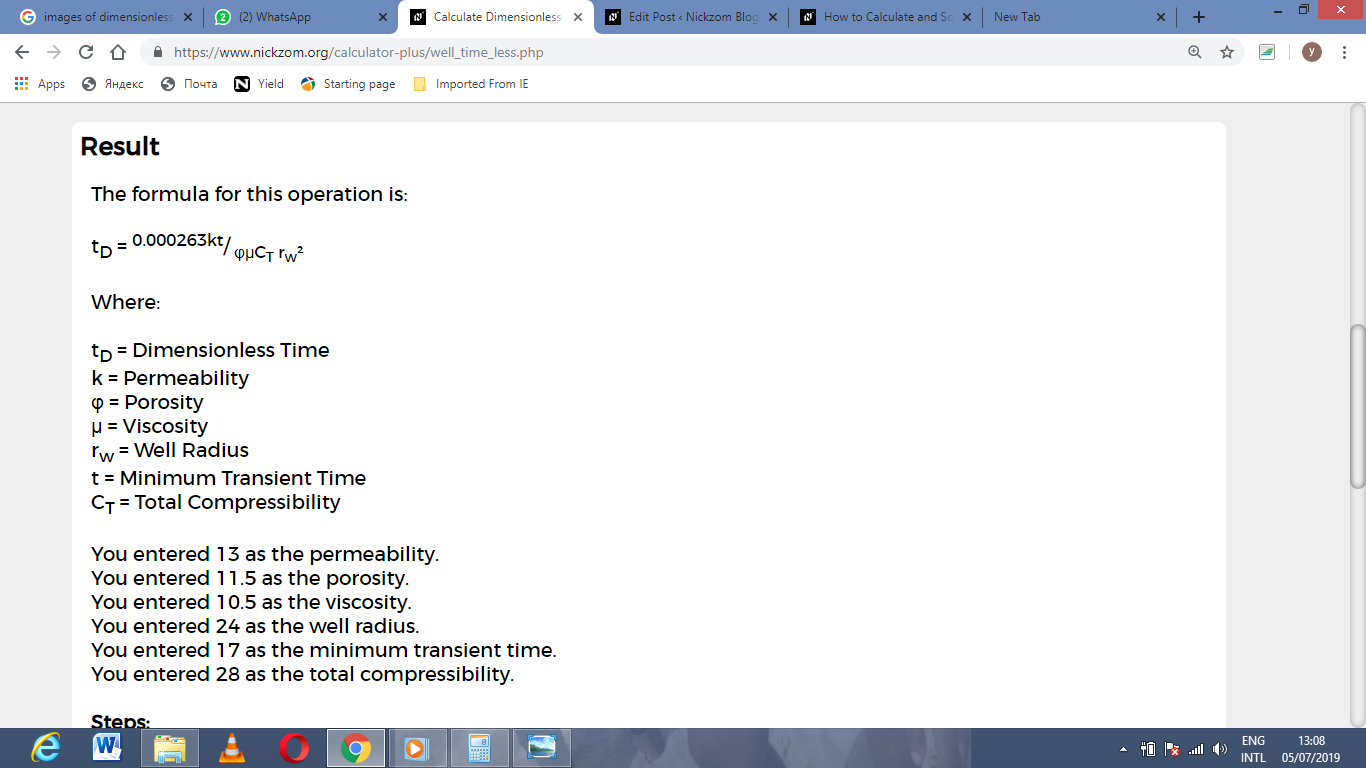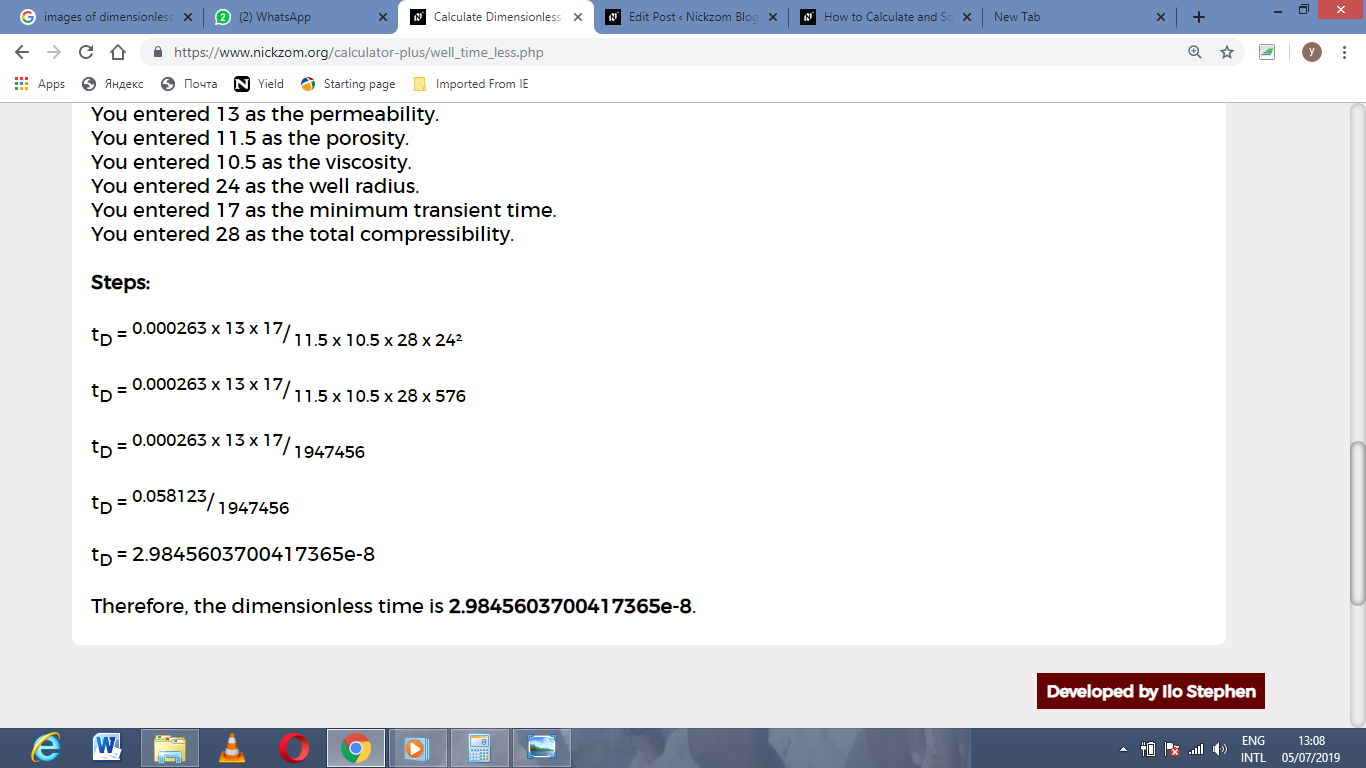As you can see from the screenshot above, Nickzom Calculator– The Calculator Encyclopedia solves for the dimensionless time and presents the formula, workings and steps too.